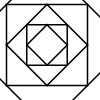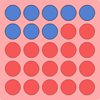#### You may also like### Tangram Tangle

If you split the square into these two pieces, it is possible to fit the pieces together again to make a new shape. How many new shapes can you make?### Shaping It

These pictures were made by starting with a square, finding the half-way point on each side and joining those points up. You could investigate your own starting shape.### All Change

There are three versions of this challenge. The idea is to change the colour of all the spots on the grid. Can you do it in fewer throws of the dice?

# Weighted Numbers

## Weighted Numbers

Full Screen Version

Here is a balance. It has a hook on each end from which you can hang weights.

My weights are numbers - I have $1$s, $2$s, $3$s, $4$s, $5$s, $6$s, $7$s, $8$s, $9$s and $10$s.

If I put a $2$ on one side and two $1$s on the other side, it is balanced.
If I put a $3$ on one side, I would need three $1$s to make it balance. How else could I make it balance with just a $3$ on one side?

If I had a $3$ and a $4$ on one side and three $2$s on the other side, what do I need to add to make it balance and where?

I hang a $10$ on one side. How many different ways can you find of hanging numbers on the other side to make it balance?

### Why do this problem?

This problem provides a novel context in which children can practise number bonds. Encouraging learners to articulate the conditions for when the numbers will be balanced is a fantastic opportunity to assess their numerical understanding and to help them use appropriate mathematical vocabulary.

### Possible approach

Pupils will need practical experience of exploring balances before trying this problem. A number of simple balances for comparing weights are available from different suppliers. Unfortunately the toy that inspired our creation of this problem seems to have been withdrawn from sale but the idea can be conveyed using any balance scales.

You could introduce this problem by showing the interactivity to the class. You could put a $2$ weight on one side of the balance and then two $1$ weights on the other without saying anything. Ask the children to describe what is happening. Now put a $3$ weight on one side and two $1$s on the other and invite the children to comment on what you must do in order to balance the equaliser. Invite a child to test the ideas.

You may want the children to be at a computer in pairs or you may prefer them to work on mini-whiteboards/paper to try the rest of the problem. After leaving time for them to work, bring them together and discuss their solutions. You may want to ask pairs to write up some ways of balancing $10$ on individual strips of paper which can be stuck on the board. You could follow on by looking for patterns in the solutions, which may lead to the children suggesting other possibilities which have been left out. Finding all the possible combinations is very hard at this level, so you could leave space on the wall for children to add others over an extended period of time.

Alternatively (or in addition) you could focus on the number sentences that can be written from the pupils' solutions.

### Key questions

Can you describe how the balance works?
Are there any other ways of making it balance?